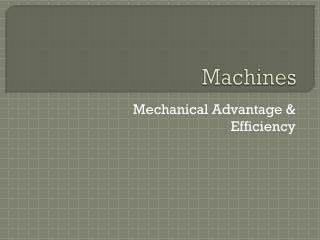DownloadDownload PresentationMachines

# Machines

Télécharger la présentation## Machines

- - - - - - - - - - - - - - - - - - - - - - - - - - - E N D - - - - - - - - - - - - - - - - - - - - - - - - - - -
##### Presentation Transcript

1. Machines Mechanical Advantage & Efficiency

2. Mechanical Advantage 3m 1m FE= ?? FL = 30 N * Using the law of levers to solve. ∴ The effort force required is 3 times less! Consider the following first class lever, what force is required for static eqm?

3. In general, using the law of levers: • We have two ratios in the equation which tell us about mechanical advantages: * Rearranging for ratios Actual Mechanical Advantage Ideal Mechanical Advantage

4. Why two advantages? • Most machines have friction due to moving parts so they are not ideal. • IMAs can be modified to suit the machine. • IMAs of Other machines:

5. Efficiency of Machines The ratio of AMA to the IMA of a machine expressed as a percentage:

6. Eg.1:Consider ateeter-totter with a load of 100N at a distance of 40cm and an effort force of 50N at a distance of 80cm. Calculate • The AMA & IMA • The %eff • Eg.2: A 25-N cart is pulled 2m up a ramp with an effort force of 6N parallel to the ramp raising the cart 0.6m above its initial level. • The AMA & IMA • The %eff of the ramp.